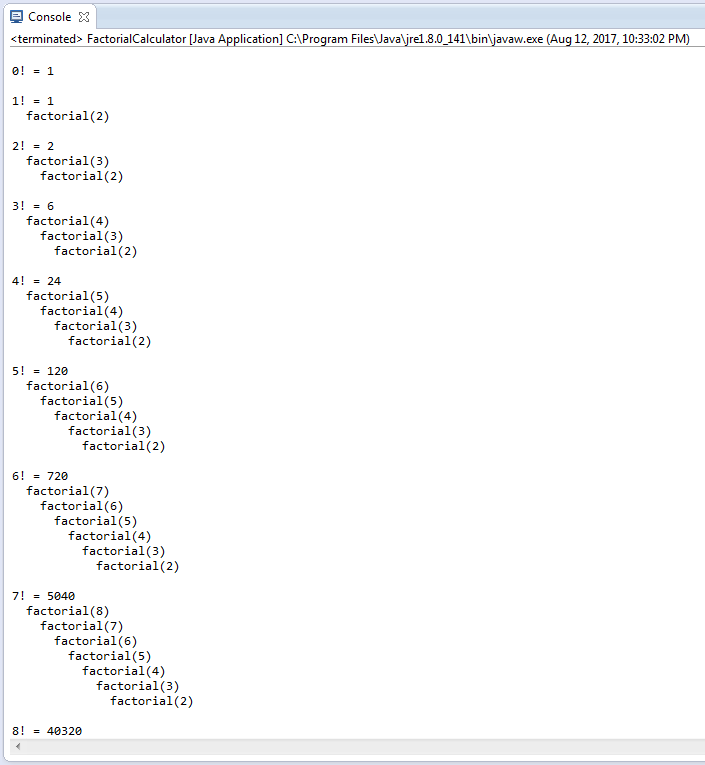# Question & Answer: It is interesting to watch recursion “in action.” Modify the factorial method in Fig. 18.3 to print its local variable and recursive-call parameter……

It is interesting to watch recursion “in action.” Modify the factorial method in Fig. 18.3 to print its local variable and recursive-call parameter.

For each recursive call, display the outputs on a separate line and add a level of indentation. Do your utmost to make the outputs clear, interesting, and meaningful. Your goal here is to design and implement an output format that makes it easier to understand recursion.

Don't use plagiarized sources. Get Your Custom Essay on
Question & Answer: It is interesting to watch recursion “in action.” Modify the factorial method in Fig. 18.3 to print its local variable and recursive-call parameter……
GET AN ESSAY WRITTEN FOR YOU FROM AS LOW AS \$13/PAGE
```// Fig. 18.3: FactorialCalculator.java
// Recursive factorial method.

public class FactorialCalculator
{
// recursive method factorial (assumes its parameter is >= 0
public static long factorial(long number)
{
if (number <= 1) // test for base case
return 1; // base cases: 0! = 1 and 1! = 1
else // recursion step
return number * factorial(number - 1);
}

// output factorials for values 0-21
public static void main(String[] args)
{
// calculate the factorials of 0 through 21
for (int counter = 0; counter <= 21; counter++)
System.out.printf("%d! = %d%n", counter, factorial(counter));
}
} // end class FactorialCalculator
```

public class FactorialCalculator {

// we will store the depth of recursion in below variable

static int level = 0;

// recursive method factorial (assumes its parameter is >= 0

public static long factorial(long number) {

if (number <= 1) { // test for base case

System.out.println();

// reset the depth of recusrion for other cases

level = 0;

return 1; // base cases: 0! = 1 and 1! = 1

}else {

// print some spaces according to depth of recursion

for(int i=0; i<=level; i++) {

System.out.print(” “);

}

System.out.println(“factorial(” + number + “)”);

// increment depth of recusrion

level++;

// recursion step

return number * factorial(number – 1);

}

}

// output factorials for values 0-21

public static void main(String[] args) {

// calculate the factorials of 0 through 21

for (int counter = 0; counter <= 21; counter++)

System.out.printf(“%d! = %d%n”, counter, factorial(counter));

}

}